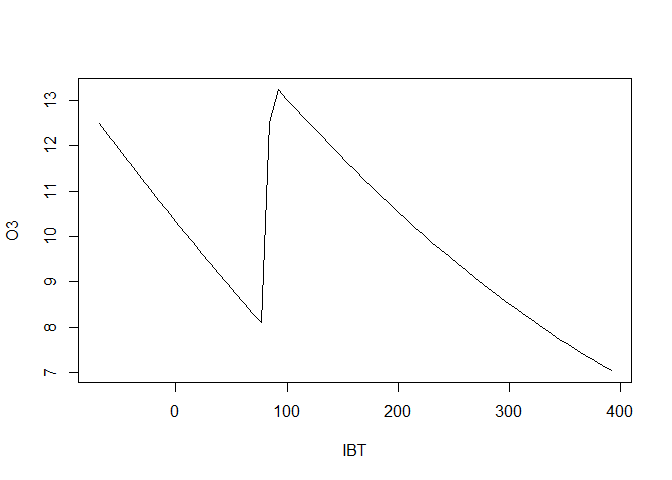# Neural networks with the nnet package

This example is based on one from Faraway (2016) “Extending the linear model with R” starting on page 368 of the book (pdf page 384). This is a pretty basic example. Much more sophisticated models are now available. However, it is a useful place to start as you learn to apply neural network models.

The ozone data set: A study the relationship between atmospheric ozone concentration and meteorology in the Los Angeles, California area in 1976. 330 observation on 10 variables:

• O3 = Ozone concentration (ppm) at Sandbug Airforce Base
• vh = a numeric vector
• wind = wind speed
• humidity
• temp = temperature measured at El Monte
• ibh = inversion base height at LAX airport
• dpg = Daggett pressure gradient
• ibt = inversion top temperature at LAX
• vis = visibility
• doy = day of the year (33 to 390… I’m not sure what the 390th day of the year means)
data(ozone, package="faraway")
head(ozone)
##   O3   vh wind humidity temp  ibh dpg ibt vis doy
## 1  3 5710    4       28   40 2693 -25  87 250  33
## 2  5 5700    3       37   45  590 -24 128 100  34
## 3  5 5760    3       51   54 1450  25 139  60  35
## 4  6 5720    4       69   35 1568  15 121  60  36
## 5  4 5790    6       19   45 2631 -33 123 100  37
## 6  4 5790    3       25   55  554 -28 182 250  38
dim(ozone)
##  330  10
#EDA
ggplot(ozone, aes(x=temp, y=O3)) + geom_point(size=1) + geom_smooth()
## geom_smooth() using method = 'loess' and formula 'y ~ x'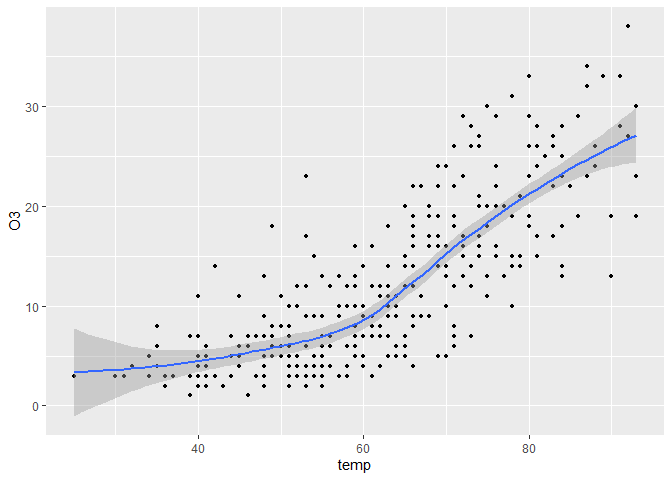ggplot(ozone, aes(x=ibh, y=O3)) + geom_point(size=1) + geom_smooth() +
theme(axis.text.x = element_text(angle = 90))
## geom_smooth() using method = 'loess' and formula 'y ~ x'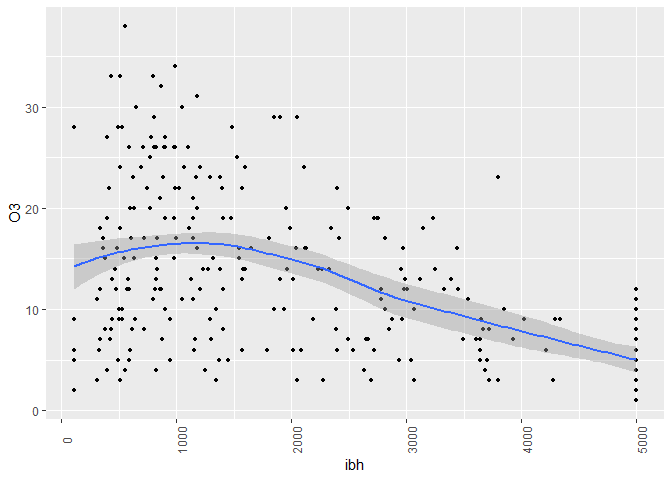ggplot(ozone, aes(x=ibt, y=O3)) + geom_point(size=1) + geom_smooth()
## geom_smooth() using method = 'loess' and formula 'y ~ x'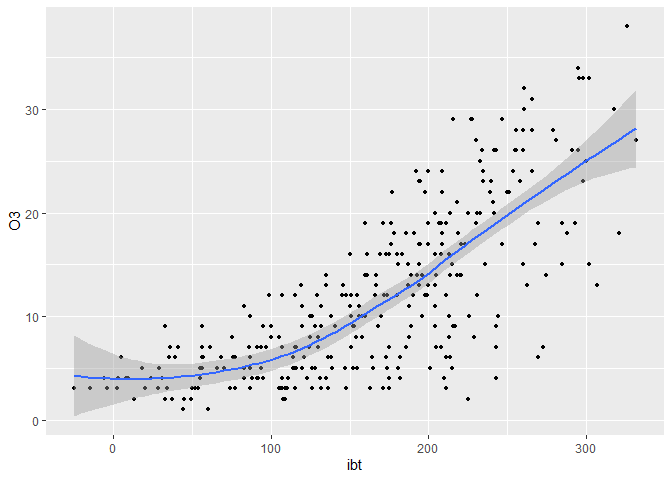###Neural nets are pretty useless when the data aren’t rescaled

Note: some software may rescale the data for you, but nnet does not.

set.seed(123)
nnmdl <- nnet(O3 ~ temp + ibh + ibt, ozone, size=2, linout=T)
## # weights:  11
## initial  value 61722.135446
## final  value 21115.406061
## converged
#RSS (sum (y_i - y.hat_i)^2)
nnmdl$value ##  21115.41 # compare to the naive RSS (numerator of the sd) sum((ozone$O3-mean(ozone$O3))^2) ##  21115.41 ###Rescaled data First, rescale the data to see some improvement. Then use 100 random starting points for the weights (100 epochs) and find the best fit among these. rescaled.ozone <- scale(ozone) set.seed(25373) best.rss=NULL for(i in 1:100){ nnmdl <- nnet(O3 ~ temp + ibh + ibt, data=rescaled.ozone, size=2, linout=T, trace=F) best.rss[i]=nnmdl$value
}
min(best.rss)
##  91.81211
which.min(best.rss)
##  1
plot(sort(best.rss))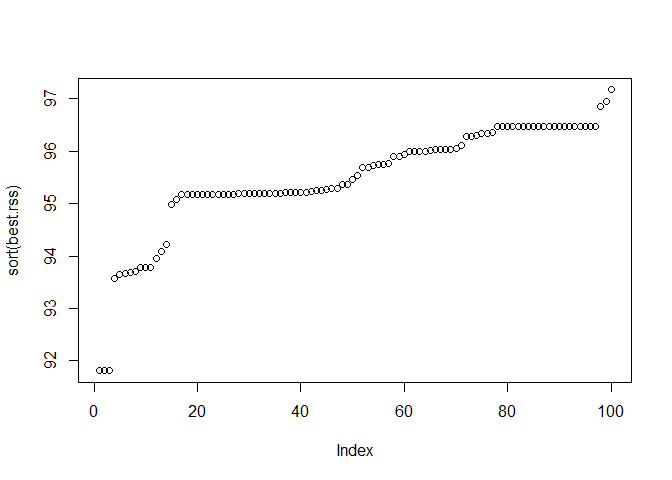sort(best.rss)
##    91.81211 91.81211 91.81211 93.57400 93.65448 93.67610 93.69477 93.69982
##    93.78377 93.78652 93.78803 93.96104 94.08442 94.21903 94.97327 95.07801
##   95.16609 95.16686 95.16927 95.17097 95.17180 95.17376 95.17561 95.17662
##   95.17711 95.17793 95.17822 95.18333 95.18520 95.18768 95.18898 95.19153
##   95.19307 95.19334 95.19429 95.19804 95.20303 95.20444 95.20519 95.21170
##   95.21961 95.22401 95.24774 95.25222 95.26241 95.28337 95.28989 95.35380
##   95.35973 95.46366 95.53064 95.68703 95.69349 95.73292 95.73559 95.74324
##   95.75644 95.88942 95.89484 95.93702 95.98442 95.98515 95.98516 95.98537
##   96.01907 96.02942 96.03438 96.03599 96.03739 96.05264 96.09890 96.26944
##   96.27747 96.28751 96.33104 96.34394 96.35294 96.47215 96.47215 96.47215
##   96.47215 96.47215 96.47215 96.47215 96.47215 96.47215 96.47215 96.47215
##   96.47215 96.47215 96.47215 96.47215 96.47215 96.47216 96.47216 96.47218
##   96.47221 96.85753 96.94571 97.16413

Note that another random seed gives a slightly better minimum RSS

set.seed(25374)
for(i in 1:100){
nnmdl <- nnet(O3 ~ temp + ibh + ibt,
data=rescaled.ozone, size=2, linout=T, trace=F)
best.rss[i]=nnmdl$value if(nnmdl$value < bestrss){
bestnn <- nnmdl
bestrss <- nnmdl$value } } min(best.rss) ##  88.14799 which.min(best.rss) ##  13 Note that the naive RSS= 21,115 is a lot higher than the best neural net RSS found above of 88. We can never know whether we found the global minimum. The model has 11 weights (parameters). The plot above clearly suggests that there are many local minima. By trying many random starts for the weights, we can improve the chances that we found a good stopping point. Now, let’s examine the best model that we found. bestnn ## a 3-2-1 network with 11 weights ## inputs: temp ibh ibt ## output(s): O3 ## options were - linear output units summary(bestnn) ## a 3-2-1 network with 11 weights ## options were - linear output units ## b->h1 i1->h1 i2->h1 i3->h1 ## -1.11 0.94 -0.84 -0.28 ## b->h2 i1->h2 i2->h2 i3->h2 ## -72.32 35.15 -130.26 -70.70 ## b->o h1->o h2->o ## -1.15 4.47 -0.69 ##Interpreting nnet output ozmeans <- colMeans(ozone) ozscales <- apply(ozone,2,sd) xx <- expand.grid(temp=seq(-3,3,0.1),ibh=0,ibt=0) plot(xx$temp*ozscales["temp"]+ozmeans["temp"],
predict(bestnn,new=xx)*ozscales["O3"]+ozmeans["O3"],
xlab="Temp", ylab="O3", type="l")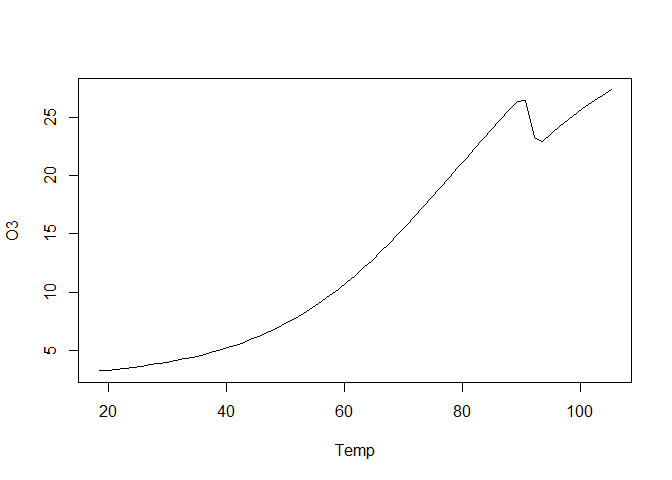xx <- expand.grid(temp=0,ibh=seq(-3,3,0.1),ibt=0)
plot(xx$ibh*ozscales["ibh"]+ozmeans["ibh"], predict(bestnn,new=xx)*ozscales["O3"]+ozmeans["O3"], xlab="IBH", ylab="O3", type="l")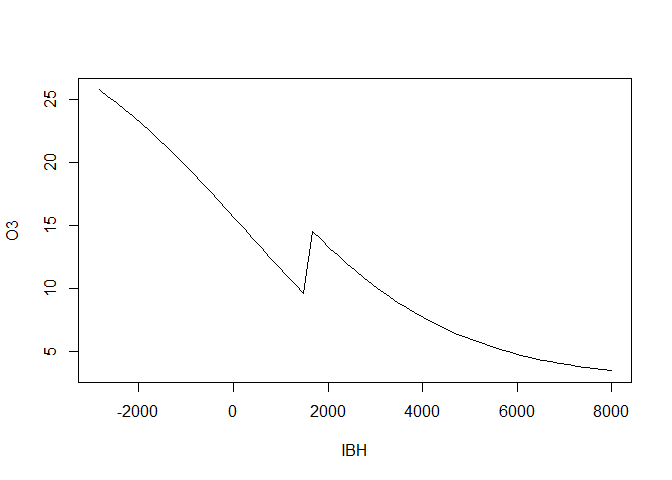xx <- expand.grid(temp=0,ibh=0,ibt=seq(-3,3,0.1)) plot(xx$ibt*ozscales["ibt"]+ozmeans["ibt"],
predict(bestnn,new=xx)*ozscales["O3"]+ozmeans["O3"],
xlab="IBT",ylab="O3", type="l")